Published

# High/Low Temperature Sensor

We have constructed a temperature sensor to alert at high and low temperatures with a buzzer.

BeginnerShowcase (no instructions)572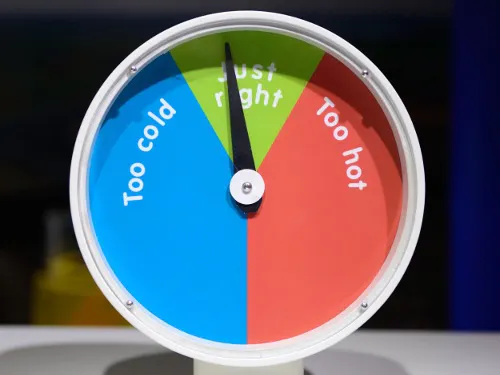## Things used in this project

### Hardware componentsParticle Photon
×2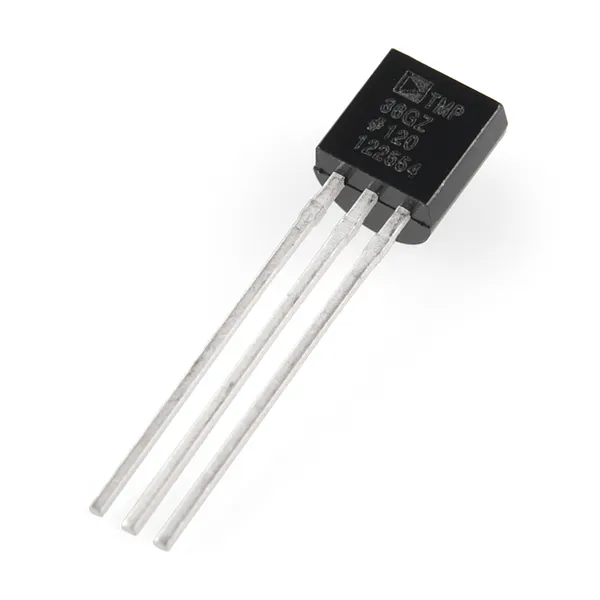Temperature Sensor Probe Temp Sensor from Particle Photon Kit
×1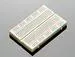×2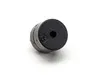Buzzer Buzzer from Particle Photon Kit
×1Jumper wires (generic)
×1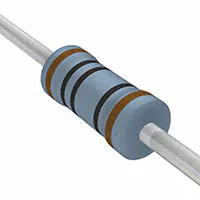Resistor 100 ohm
×1

## Schematics

### Temp Sensor Circuit (Schematic)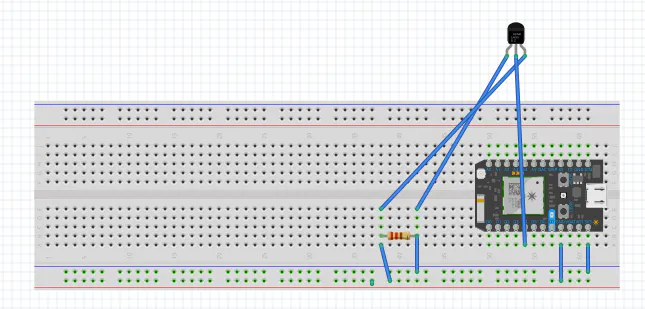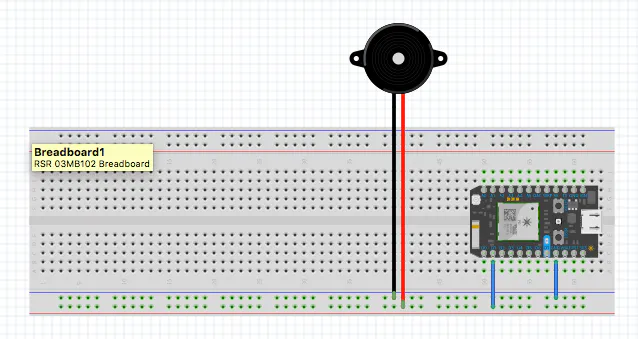### Temp Sensor Circuit (Actual)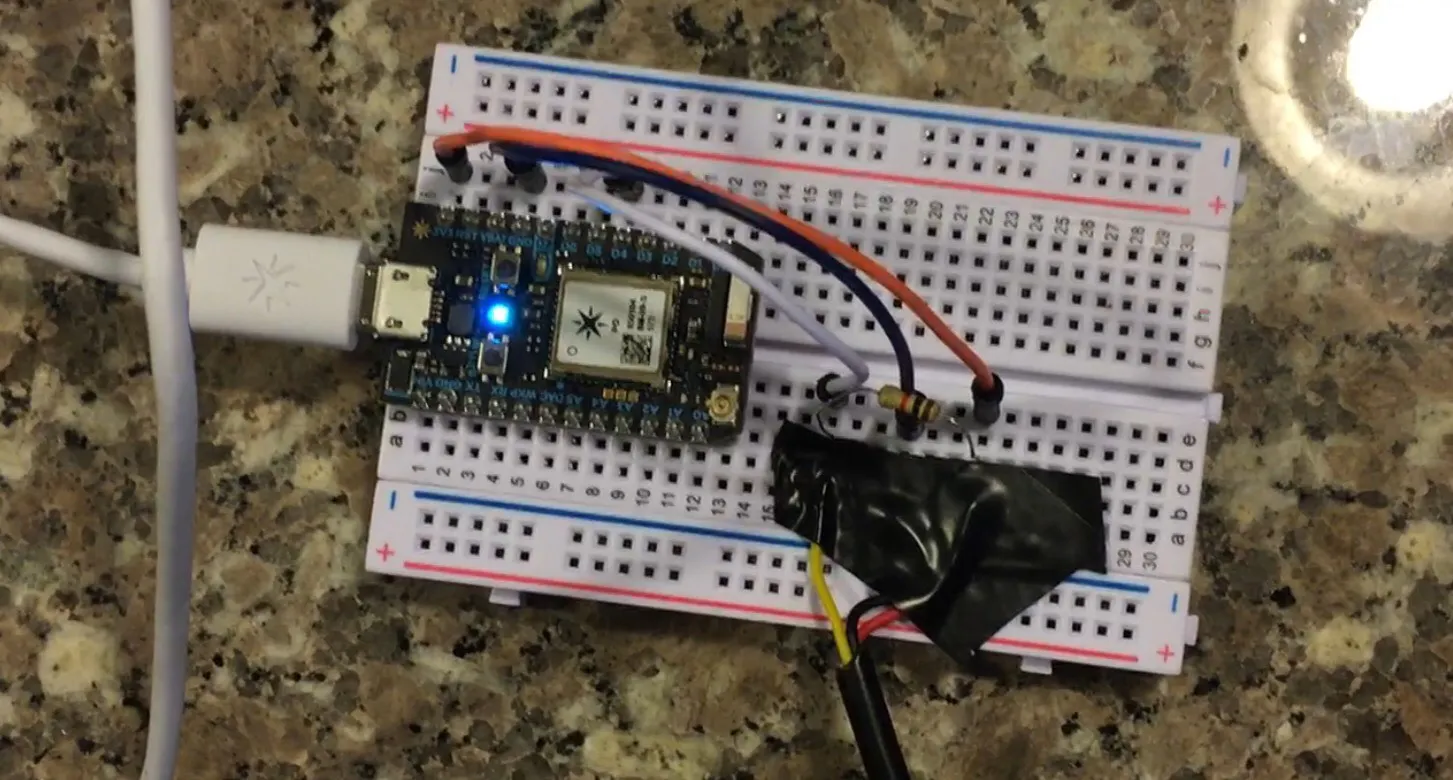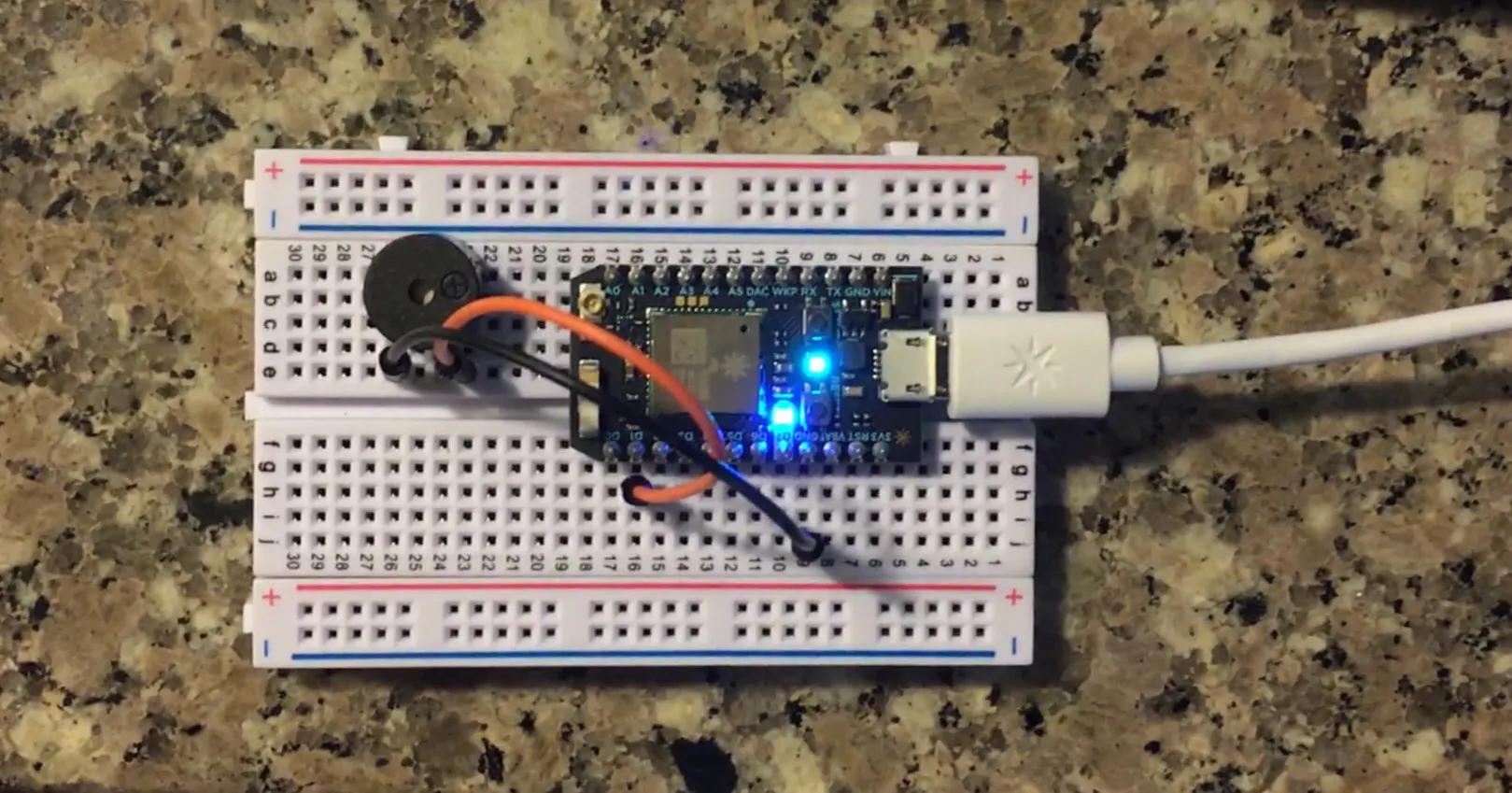### screen_shot_2017-04-12_at_8_06_38_pm_QqmeHYpXCg.png

Google Docs Chart of Engine Bay Temp vs. Time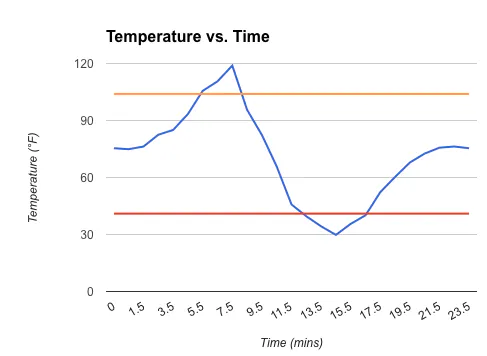## Code

### Temperature Sensor Code

C/C++
```OneWire ds = OneWire(D4);  // 1-wire signal on pin D4

unsigned long lastUpdate = 0;

float lastTemp;

void setup() {
Serial.begin(9600);
// Set up 'power' pins, comment out if not used!
pinMode(D3, OUTPUT);
pinMode(D5, OUTPUT);
digitalWrite(D3, LOW);
digitalWrite(D5, HIGH);

}

// up to here, it is the same as the address acanner
// we need a few more variables for this example

void loop(void) {
byte i;
byte present = 0;
byte type_s;
byte data;
float celsius, fahrenheit;

Serial.println();
ds.reset_search();
delay(250);
return;
}

// The order is changed a bit in this example
// first the returned address is printed

Serial.print("ROM =");
for( i = 0; i < 8; i++) {
Serial.write(' ');
}

// second the CRC is checked, on fail,

Serial.println("CRC is not valid!");
return;
}
Serial.println();

// we have a good address at this point
// what kind of chip do we have?
// we will set a type_s value for known types or just return

// the first ROM byte indicates which chip
case 0x10:
Serial.println("  Chip = DS1820/DS18S20");
type_s = 1;
break;
case 0x28:
Serial.println("  Chip = DS18B20");
type_s = 0;
break;
case 0x22:
Serial.println("  Chip = DS1822");
type_s = 0;
break;
case 0x26:
Serial.println("  Chip = DS2438");
type_s = 2;
break;
default:
Serial.println("Unknown device type.");
return;
}

// this device has temp so let's read it

ds.reset();               // first clear the 1-wire bus
ds.select(addr);          // now select the device we just found
// ds.write(0x44, 1);     // tell it to start a conversion, with parasite power on at the end
ds.write(0x44, 0);        // or start conversion in powered mode (bus finishes low)

// just wait a second while the conversion takes place
// different chips have different conversion times, check the specs, 1 sec is worse case + 250ms
// you could also communicate with other devices if you like but you would need

delay(1000);     // maybe 750ms is enough, maybe not, wait 1 sec for conversion

// we might do a ds.depower() (parasite) here, but the reset will take care of it.

// first make sure current values are in the scratch pad

present = ds.reset();
ds.write(0xB8,0);         // Recall Memory 0
ds.write(0x00,0);         // Recall Memory 0

present = ds.reset();
if (type_s == 2) {
ds.write(0x00,0);       // The DS2438 needs a page# to read
}

// transfer and print the values

Serial.print("  Data = ");
Serial.print(present, HEX);
Serial.print(" ");
for ( i = 0; i < 9; i++) {           // we need 9 bytes
Serial.print(data[i], HEX);
Serial.print(" ");
}
Serial.print(" CRC=");
Serial.print(OneWire::crc8(data, 8), HEX);
Serial.println();

// Convert the data to actual temperature
// because the result is a 16 bit signed integer, it should
// be stored to an "int16_t" type, which is always 16 bits
// even when compiled on a 32 bit processor.
int16_t raw = (data << 8) | data;
if (type_s == 2) raw = (data << 8) | data;
byte cfg = (data & 0x60);

switch (type_s) {
case 1:
raw = raw << 3; // 9 bit resolution default
if (data == 0x10) {
// "count remain" gives full 12 bit resolution
raw = (raw & 0xFFF0) + 12 - data;
}
celsius = (float)raw * 0.0625;
break;
case 0:
// at lower res, the low bits are undefined, so let's zero them
if (cfg == 0x00) raw = raw & ~7;  // 9 bit resolution, 93.75 ms
if (cfg == 0x20) raw = raw & ~3; // 10 bit res, 187.5 ms
if (cfg == 0x40) raw = raw & ~1; // 11 bit res, 375 ms
// default is 12 bit resolution, 750 ms conversion time
celsius = (float)raw * 0.0625;
break;

case 2:
data = (data >> 3) & 0x1f;
if (data > 127) {
celsius = (float)data - ((float)data * .03125);
}else{
celsius = (float)data + ((float)data * .03125);
}
}

// remove random errors
if((((celsius <= 0 && celsius > -1) && lastTemp > 5)) || celsius > 125) {
celsius = lastTemp;
}

fahrenheit = celsius * 1.8 + 32.0;
lastTemp = celsius;
Serial.print("  Temperature = ");
Serial.print(celsius);
Serial.print(" Celsius, ");
Serial.print(fahrenheit);
Serial.println(" Fahrenheit");

if(lastTemp < 5) {
String temperature = String(fahrenheit);
Particle.publish("Engine_Bay_Temp_Low!", temperature);
delay(30000);
}

if(lastTemp > 40) {
String temperature = String(fahrenheit);
Particle.publish("Engine_Bay_Temp_High!", temperature);
delay(30000);
}

// now that we have the readings, we can publish them to the cloud
String temperature = String(fahrenheit); // store temp in "temperature" string
Particle.publish("Engine_Bay_Temp", temperature); // publish to cloud
delay(30000);

}

```

C/C++
```int led = D7;

void setup()
{
pinMode(D1,OUTPUT);
pinMode(D7,OUTPUT);
digitalWrite(led,LOW);
Particle.subscribe("Engine_Bay_Temp_Low!", temperature, "myHandler");
Particle.subscribe("Engine_Bay_Temp_High!", temperature, "myHandler");
}

void temperature(const char *event, const char *data)
{

if ("Engine_Bay_Temp_High!")
digitalWrite(led,HIGH);

else
digitalWrite(led,LOW);

if (strcmp(data,"Engine_Bay_Temp_Low!"))
{
digitalWrite(D1,HIGH);delay(300);digitalWrite(D1,LOW);delay(200);digitalWrite(D1,HIGH);delay(100);digitalWrite(D1,LOW);delay(300);digitalWrite(D1,HIGH);
delay(200);digitalWrite(D1,LOW);delay(100);digitalWrite(D1,HIGH);delay(300);digitalWrite(D1,LOW);delay(200);digitalWrite(D1,HIGH);delay(100);digitalWrite(D1,LOW);
delay(300);digitalWrite(D1,HIGH);delay(200);digitalWrite(D1,LOW);delay(100);digitalWrite(D1,HIGH);delay(300);digitalWrite(D1,LOW);delay(2000);

digitalWrite(D1,HIGH);delay(300);digitalWrite(D1,LOW);delay(200);digitalWrite(D1,HIGH);delay(100);digitalWrite(D1,LOW);delay(300);digitalWrite(D1,HIGH);
delay(200);digitalWrite(D1,LOW);delay(100);digitalWrite(D1,HIGH);delay(300);digitalWrite(D1,LOW);delay(200);digitalWrite(D1,HIGH);delay(100);digitalWrite(D1,LOW);
delay(300);digitalWrite(D1,HIGH);delay(200);digitalWrite(D1,LOW);delay(100);digitalWrite(D1,HIGH);delay(300);digitalWrite(D1,LOW);delay(2000);

digitalWrite(D1,HIGH);delay(300);digitalWrite(D1,LOW);delay(200);digitalWrite(D1,HIGH);delay(100);digitalWrite(D1,LOW);delay(300);digitalWrite(D1,HIGH);
delay(200);digitalWrite(D1,LOW);delay(100);digitalWrite(D1,HIGH);delay(300);digitalWrite(D1,LOW);delay(200);digitalWrite(D1,HIGH);delay(100);digitalWrite(D1,LOW);
delay(300);digitalWrite(D1,HIGH);delay(200);digitalWrite(D1,LOW);delay(100);digitalWrite(D1,HIGH);delay(300);digitalWrite(D1,LOW);delay(2000);

digitalWrite(D1,HIGH);delay(300);digitalWrite(D1,LOW);delay(200);digitalWrite(D1,HIGH);delay(100);digitalWrite(D1,LOW);delay(300);digitalWrite(D1,HIGH);
delay(200);digitalWrite(D1,LOW);delay(100);digitalWrite(D1,HIGH);delay(300);digitalWrite(D1,LOW);delay(200);digitalWrite(D1,HIGH);delay(100);digitalWrite(D1,LOW);
delay(300);digitalWrite(D1,HIGH);delay(200);digitalWrite(D1,LOW);delay(100);digitalWrite(D1,HIGH);delay(300);digitalWrite(D1,LOW);delay(2000);

digitalWrite(D1,HIGH);delay(300);digitalWrite(D1,LOW);delay(200);digitalWrite(D1,HIGH);delay(100);digitalWrite(D1,LOW);delay(300);digitalWrite(D1,HIGH);
delay(200);digitalWrite(D1,LOW);delay(100);digitalWrite(D1,HIGH);delay(300);digitalWrite(D1,LOW);delay(200);digitalWrite(D1,HIGH);delay(100);digitalWrite(D1,LOW);
delay(300);digitalWrite(D1,HIGH);delay(200);digitalWrite(D1,LOW);delay(100);digitalWrite(D1,HIGH);delay(300);digitalWrite(D1,LOW);
}

else
{
delay(100);
}
}
```